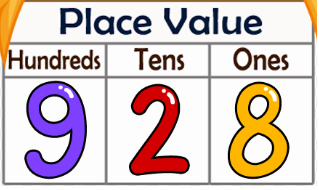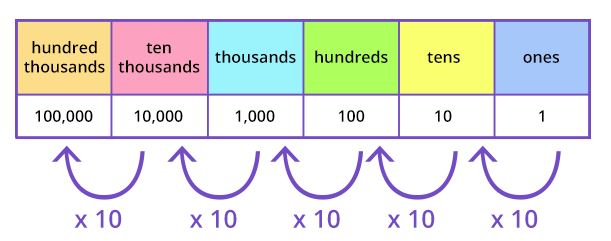Place value refers to the value of each digit in a number. For example, the number 928 has three “places”—or columns—each with a specific value. In this three-digit number, the 8 is in the “ones” place, the 2 is in the “tens” place, and the 9 is in the “hundreds” place.

Place value chart helps your kids to explore numbers from one to millions. A place-value chart is a way to make sure digits are in the correct places.

Each digit in a whole number has a place value, based on its position from the right, as seen in the following place value chart. The value of a digit in the number is the digit multiplied by the place value.  Each place on a chart has a value that is 10 times the value of the place on its right.The number 928 can be written in expanded form as:

##### 928 = 9 x 100 + 2 x 10 + 8 = 900 + 20 + 8
Categories: Math Zone